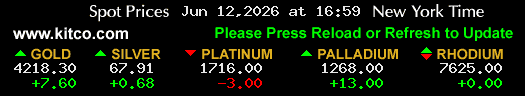Enter Weight

Grams (g)

Troy Oz. Pure Gold

0.0000

Gross Melt Value

\$ 0.00

Profit \ Loss

+\- %

P\L Value

\$ 0.00

Note: The grid view feaure is only available for Pro members.

### Gold Valuation Tools:

1. Gold Testing Kit. Want to know if your gold is real? A simple acid test will give you confirmation.

2. Digital Scale. When weighing gold you want to be as precise as possible. We recommend a scale that is accurate to .01 or 1/100 gram.

USD

#### 10 Karat

 1 gram = 21.07 1 .dwt = 32.77 5 grams = 105.35 20 grams = 421.40 1 troy oz. = 655.36

#### 14 Karat

 1 gram =29.46 1 .dwt =45.81 5 grams =147.29 20 grams =589.16 1 troy .oz =916.24

#### 18 Karat

 1 gram =37.90 1 .dwt =58.94 5 grams =189.48 20 grams =757.92 1 troy .oz =1,178.70

#### 22 Karat

 1 gram =46.33 1 .dwt =72.06 5 grams =231.67 20 grams =926.69 1 troy .oz =1,441.16

USD

1 gram

50.53

2.5 grams

126.32

.10 troy .oz

157.16

5 grams

252.64

.25 troy .oz

392.90

10 grams

505.28

.5 troy .oz

785.80

20 grams

1,010.56

50 grams

2,526.41

100 grams

5,052.81